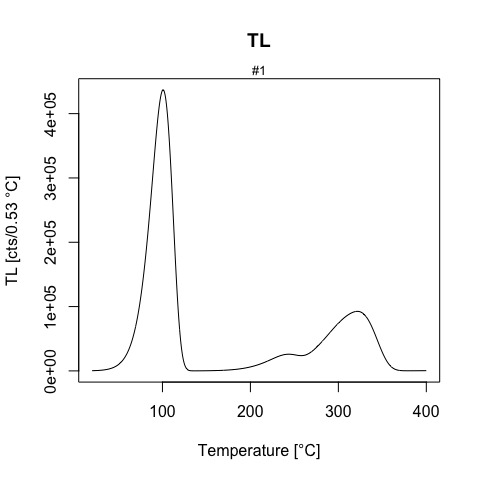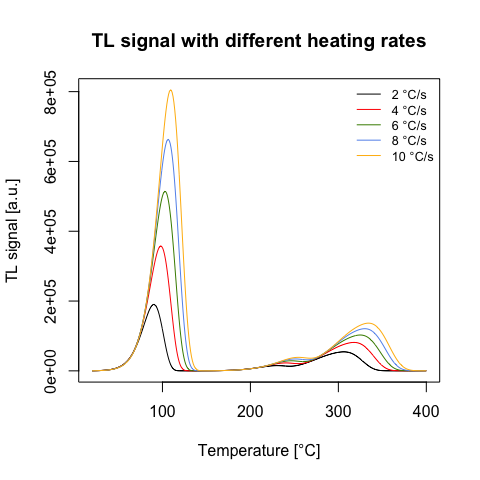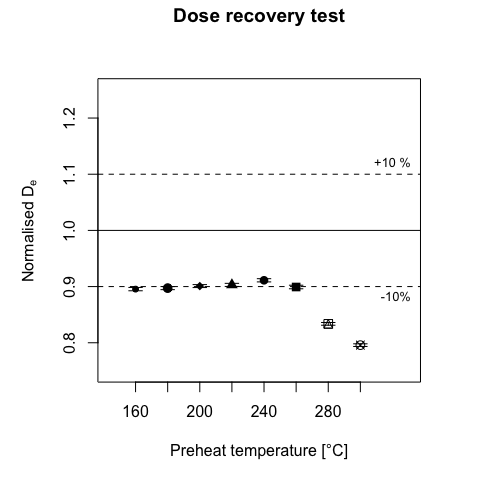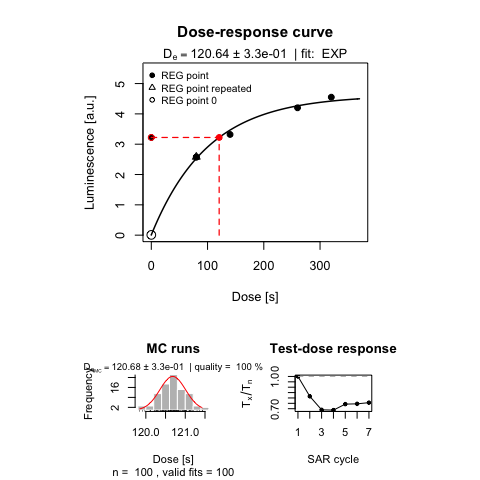# 1 Introduction

This vignette shows a few examples for the R-package ‘RLumModel.’ The main function model_LuminescenceSignals() and their arguments will be explained. All calculations were done with ‘RLumModel’ (version: 0.2.8.9000.5) and ‘Luminescence’ (version: 0.9.11.9000.30).

# 2 Object structure of RLumModel

The output from the main function model_LuminescenceSignals() is of class RLum.Analysis (Kreutzer et al. 2012) and contains data of class RLum.Data.Curve in the slot ‘records.’ The advantage of this infrastructure is that the package ‘Luminescence’ offers a lot of methods to visualize and manipulate data.

All simulated data are stored in the slot ‘records’: TL/OSL/RF curves as well as the concentrations of every energy level from every step.

The following code loads a data set provided by the ‘RLumModel’ package and shows how to separate TL/OSL/RF data from concentrations and how to visualize them.

data("ExampleData.ModelOutput", package = "RLumModel")

##show class
class(model.output)

##show structure
Luminescence::structure_RLum(model.output)

##separate TL-curve from TL-concentrations
TL_curve <- Luminescence::get_RLum(model.output, recordType = "TL$") TL_conc <- Luminescence::get_RLum(model.output, recordType = "(TL)", drop = FALSE) ##also possible: TL_curve <- get_RLum(model.output, record.id = 1) ##plot results Luminescence::plot_RLum(TL_curve) Luminescence::plot_RLum(TL_conc) Some notes to the code example above: • in ‘TL_curve <- …’ appears “TL$.” This is necessary to match the pattern “TL” without any sign after “TL,” e.g. a bracket. The brackets are used (by default) for the concentrations.
• in ‘TL_conc <- …’ the pattern “(TL)” will match all concentrations with “(TL),” see structure.
• drop = FALSE was used to keep the RLum.Analysis class for ‘TL_conc.’
• To see a single plot of every energy-level, use the option plot.single = TRUE in plot_RLum(). For more details see the manual of ‘Luminescence.’
##plot every energy-level by an extra plot
Luminescence::plot_RLum(TL_conc, plot.single = TRUE)

It is also possible to choose a RLum.Data.Curve by their ‘record.id,’ which can be seen with:

##see structure of model.output
Luminescence::structure_RLum(model.output)

# 3 Selecting a quartz luminescence model

The first argument required for the function model_LuminescenceSignals() is the name of a quartz luminescence model to be used, respectively the used parameter set in this quartz luminescence model. All currently implemented quartz luminescence models were described in Friedrich, Kreutzer, and Schmidt (2016). The command to select a set of parameters from a specific model in RLumModel is a character string with the name of the author and the year, e.g.

model <- "Bailey2001"

The available models are “Bailey2001,” “Bailey2002,” “Bailey2004,” “Pagonis2007,” “Pagonis2008,”“Friedrich2017” and “customized” (R. M. Bailey (2001), R. Bailey (2002), R. Bailey (2004), V. Pagonis, Chen, and Wintle (2007), V. Pagonis et al. (2008a), Friedrich et al. (2017)). For customized or own parameter sets, see vignette RLumModel - Using own parameter sets

The corresponding parameter set will be loaded automatically with the function call.

# 4 Creating a sequence

The second argument in the model_LuminescenceSignals() function is the sequence to be simulated. There are three different ways of creating a sequence.

For all sequences, temperature differences between sequence steps are automatically simulated with a heating or cooling step in between. Also, after irradiating the sample, it is automatically kept at irradiation temperature for further 5 s to allow the system to relax prior to the next step (R. M. Bailey 2001).

## 4.1 Risø SEQ files

The first one is to use the popular and freely available Risø to build a personal sequence and to save it as a SEQ-file (*.seq). Files created by the Sequence Editor can be imported directly using the path of the SEQ-file. The package comes along with an example SEQ-file in the package folder in ‘extdata.’ Thus, a potential sequence is created with

sequence <- system.file(
"extdata",
"example_SAR_cycle.SEQ",
package = "RLumModel")

or wherever the SEQ-file is stored. While in the Sequence Editor irradiation is commonly defined in seconds, performing the simulation requires a dose transformation to Gray. Therefore, the function model_LuminescenceSignals() offers a special argument called lab.dose_rate, representing the dose rate of the irradiation unit in the laboratory. By default, this dose rate is 1 Gy/s, but can be modified, e.g.,

lab.dose_rate <-  0.105

## 4.2 Keywords

The second way of creating a sequence is by referring to a list with keywords and a certain order of code numbers or named values, which are shown in Table 1. With these keywords, it is possible to create quickly an R object of type list, which can be read by the model_LuminescenceSignals() function.

Keywords in RLumModel for creating sequences
ARGUMENTS DESCRIPTION SUB-ARGUMENTS
TL Thermally stimulated luminescence ’temp_begin’ [°C], ’temp_end’ [°C], ’heating_rate’ [°C/s]
OSL Optically stimulated luminescence ’temp’ [°C], ’duration’ [s], ’optical_power’ [%]
ILL Illumination ’temp’ [°C], ’duration’ [s], ’optical_power’ [%]
LM_OSL Linear modulated OSL ’temp’ [°C], ’duration’ [s], optional: ’start_power’ [%], ’end_power’ [%]
RF Radiofluorescence ’temp’ [°C], ’dose’ [Gy], ’dose_rate’ [Gy/s]
RF_heating RF during heating/cooling ’temp_begin’ [°C], ’temp_end’ [°C], ‘heating rate’ [°C/s], ‘dose_rate’ [Gy/s]
IRR Irradiation ’temp’ [°C], ’dose’ [Gy], ’dose_rate’ [Gy/s]
CH Cutheat ’temp’ [°C], optional: ’duration’ [s], ’heating_rate’ [°C/s]
PH Preheat ’temp’ [°C], ’duration’ [s], optional: ’heating_rate’ [°C/s]
PAUSE Pause ’temp’ [°C], ’duration [s]’

Some examples to this kind of sequence creating:

sequence <- list(
IRR = c(temp = 20, dose = 10, dose_rate = 1),
TL = c(temp_begin = 20, temp_end = 400 , heating_rate = 5))

This sequences describes an irradiation simulation at 20 °C with a dose of 10 Gy and a dose rate of 1 Gy/s, which is followed by a TL simulation from 20 °C to 400 °C with a heating rate of 5 °C/s. Note that it is important that for each sequence keyword like ‘IRR’ or ‘TL’ either the vector has to be named or the correct order of arguments is used, see ‘sub-arguments’ in Table 1. Thus the above mentioned code is equivalent to the following one:

sequence <- list(
IRR = c(20, 10, 1),
TL = c(20, 400, 5))

## 4.3 Creating a SAR/DRT sequence

However, to create a SAR or dose-recovery-test (DRT) sequence with the Risø Sequence Editor or with keywords is time-consuming, because it contains a lot of individual sequence steps (preheat, optical stimulation, irradiation, …). Therefore, a third way was implemented in ‘RLumModel’ to create a (SAR) sequence after Murray and Wintle (2000) with the (required) keywords RegDose, TestDose, PH, CH and OSL temp. In addition to these keywords, the user is able to set more detailed parameters for the SAR sequence, see Table 2:

Keywords in RLumModel for creating SAR sequences
ABBREVIATION DESCRIPTION EXAMPLE ARGUMENTS
RegDose Dose points of the regenerative cycles [Gy] c(0, 80, 140, 260, 320, 0, 80)
TestDose Test dose for the SAR cycles [Gy] 50
PH Temperature of the preheat [°C] 240
CH Temperature of the cutheat [°C] 200
OSL_temp Temperature of OSL read out [°C] 125
OSL_duration Duration of OSL read out [s] default: 40
Irr_temp Temperature of irradiation [°C] default: 20
PH_duration Duration of the preheat [s] default: 10
dose_rate Dose rate of the laboratory irradiation source [Gy/s] default: 1
optical_power Percentage of the full illumination power [%] default: 90
Irr_2recover Dose to be recovered in a dose-recovery-test [Gy] 20

So a possible DRT sequence could be the next code example:

sequence <- list(
RegDose = c(0,10,20,50,90,0,10),
TestDose = 2,
PH = 220,
CH = 220,
OSL_temp = 125,
Irr_2recover = 20)

This sequence describes a DRT, where a dose of 20 Gy will be recovered with this test. The regenerative doses are defined as 0 (natural), 10 Gy, 20 Gy, 50 Gy, 90 Gy and for recuperation and recycling ratio 0 Gy and 10 Gy, respectively. The test dose is defined as 2 Gy. Preheat and cutheat are at 220 °C and all OSL measurements are simulated at 125 °C. There are more options to set, see Table 2.

The ‘RLumModel’ function model_LuminescenceSignals() is able to interpret this (sequence-) list as a DRT sequence.

# 5 Working examples

## 5.1 Simulate a TL measurement

First of all, a sequence is needed, which produces a TL signal after the sample has received a dose:

sequence <- list (
IRR = c (20 , 10 , 1) ,
TL = c (20 , 400 , 5))

Here, at a temperature of 20 °C a dose of 10 Gy was applied with a dose rate of 1 Gy/s followed by a TL measurement from 20 °C to 400 °C with a heating rate of 5 °C/s. Running this sequence with the model_LuminescenceSignals() function produces a model output:

model.output <- model_LuminescenceSignals(
model = "Bailey2001",
sequence = sequence,
verbose = FALSE)TL curve with parameter set ‘Bailey2001’ after 10 Gy laboratory dose

This results in a TL curve like the one published in (R. M. Bailey (2001), Fig. 1), see figure above. In a further step, it is easy to produce known TL phenomena like the shift of the TL peak with varying heating rate. For this purpose, a loop over a TL simulation changes the heating rate in each run.

##set heating rate
heating.rate <- seq(from = 2, to = 10, by = 2)

##model signals
##"verbose = FALSE" for no terminal output
## "TL$" for exact matching TL and not (TL) model.output <- lapply(heating.rate, function(x){ sequence <- list( IRR = c(20, 10, 1), TL = c(20, 400, x)) TL_data <- model_LuminescenceSignals( sequence = sequence, model = "Bailey2001", plot = FALSE, verbose = FALSE) return(Luminescence::get_RLum(TL_data, recordType = "TL$", drop = FALSE))

})

##merge output
model.output.merged <- merge_RLum(model.output)

##plot results
plot_RLum(
object = model.output.merged,
xlab = "Temperature [\u00B0C]",
ylab = "TL signal [a.u.]",
main = "TL signal with different heating rates",
legend.text = paste(heating.rate, "°C/s"),
combine = TRUE)TL signal with different heating rates

Some notes to the code above:

• the return of the lapply function is a RLum.Analysis object, because of drop = FALSE
De.error = temp$De.Error) return(out) }) ## [plot_GrowthCurve()] Fit: EXP (interpolation) | De = 179.1 | D01 = 101.51 ## [plot_GrowthCurve()] Fit: EXP (interpolation) | De = 179.46 | D01 = 101.46 ## [plot_GrowthCurve()] Fit: EXP (interpolation) | De = 180.18 | D01 = 101.4 ## [plot_GrowthCurve()] Fit: EXP (interpolation) | De = 180.6 | D01 = 101.41 ## [plot_GrowthCurve()] Fit: EXP (interpolation) | De = 182.24 | D01 = 101.44 ## [plot_GrowthCurve()] Fit: EXP (interpolation) | De = 179.85 | D01 = 102.26 ## [plot_GrowthCurve()] Fit: EXP (interpolation) | De = 166.73 | D01 = 111.51 ## [plot_GrowthCurve()] Fit: EXP (interpolation) | De = 159.15 | D01 = 161.2 ##output as data.frame for plot_DRTResults DRT.result <- as.data.frame(do.call(rbind, DRT.output)) ##plot DRT.results Luminescence::plot_DRTResults( DRT.result, preheat = PH_temp, given.dose = 200)Dose recovery test (DRT) with the parameter set of ‘Pagonis2008’ In the code above, plot = FALSE was chosen, because a single OSL plot is not necessary to analyse a SAR sequence. To calculate a De from the produced RLum.Analysis object ‘model.output,’ the function Luminescence::analyse_SAR.CWOSL() is suitable. After specifying a number of evaluation parameters (signal and background integration interval, dose points and fit function for the dose response curve) and the analysis process, the reduced data are stored in an RLum.Results object, here termed ‘results’ . A background integration interval from 301 to 401 translates to the signal from 30 s to 40 s, because a channel has the default width of 0.1 s. Accordingly, the signal integral ranges from 0.1 s to 0.7 s. ##set RegDose RegDose = c(0, 80, 140, 260, 320, 0, 80) ##set sequence sequence <- list( RegDose = RegDose, TestDose = 20, PH = 220, CH = 220, OSL_temp = 125 ) ##model model.output <- model_LuminescenceSignals( sequence = sequence, model = "Pagonis2008", plot = FALSE, verbose = FALSE ) ##analyse SAR sequence and plot only the resulting growth curve results <- Luminescence::analyse_SAR.CWOSL( model.output, signal.integral.min = 1, signal.integral.max = 7, background.integral.min = 301, background.integral.max = 401, fit.method = "EXP", dose.points = RegDose, verbose = FALSE, plot.single = c(6) )SAR protocol with the parameter set of ‘Pagonis2008’ # 6 Miscellaneous ## 6.1 Accessing applied modelling parameters Sometimes it is useful to extract the used modelling parameters. Since the output is an RLum.Analysis-object compatible with the R package ‘Luminescence,’ this can be achieved with the following code lines: Luminescence::get_RLum(model.output, info = "parms") ##$parms.N1
##  1.5e+07
##
## $parms.N2 ##  1e+07 ## ##$parms.N3
##  4e+07
##
## $parms.N4 ##  2.5e+08 ## ##$parms.N5
##  5e+10
##
## $parms.N6 ##  5e+09 ## ##$parms.N7
##  4e+09
##
## $parms.N8 ##  3e+08 ## ##$parms.N9
##  1e+10
##
## $parms.N10 ##  1.2e+12 ## ##$parms.N11
##  3e+10
##
## $parms.E1 ##  0.97 ## ##$parms.E2
##  1.55
##
## $parms.E3 ##  1.73 ## ##$parms.E4
##  1.8
##
## $parms.E5 ##  2 ## ##$parms.E6
##  1.65
##
## $parms.E7 ##  1.6 ## ##$parms.E8
##  1.43
##
## $parms.E9 ##  1.75 ## ##$parms.E10
##  5
##
## $parms.E11 ##  5 ## ##$parms.s1
##  5e+12
##
## $parms.s2 ##  5e+14 ## ##$parms.s3
##  6.5e+13
##
## $parms.s4 ##  1.5e+13 ## ##$parms.s5
##  1e+10
##
## $parms.s6 ##  6.5e+13 ## ##$parms.s7
##  5e+12
##
## $parms.s8 ##  5e+13 ## ##$parms.s9
##  5e+14
##
## $parms.s10 ##  1e+13 ## ##$parms.s11
##  1e+13
##
## $parms.A1 ##  1e-08 ## ##$parms.A2
##  1e-08
##
## $parms.A3 ##  5e-09 ## ##$parms.A4
##  5e-10
##
## $parms.A5 ##  1e-10 ## ##$parms.A6
##  1e-11
##
## $parms.A7 ##  6e-12 ## ##$parms.A8
##  5e-07
##
## $parms.A9 ##  1e-09 ## ##$parms.A10
##  1e-14
##
## $parms.A11 ##  1e-10 ## ##$parms.B1
##  0
##
## $parms.B2 ##  0 ## ##$parms.B3
##  0
##
## $parms.B4 ##  0 ## ##$parms.B5
##  0
##
## $parms.B6 ##  0 ## ##$parms.B7
##  0
##
## $parms.B8 ##  5e-09 ## ##$parms.B9
##  5e-10
##
## $parms.B10 ##  3e-10 ## ##$parms.B11
##  1e-10
##
## $parms.Th1 ##  0.75 ## ##$parms.Th2
##  0
##
## $parms.Th3 ##  6 ## ##$parms.Th4
##  4.5
##
## $parms.Th5 ##  0 ## ##$parms.Th6
##  0.01
##
## $parms.Th7 ##  0 ## ##$parms.E_th1
##  0.1
##
## $parms.E_th2 ##  0 ## ##$parms.E_th3
##  0.1
##
## $parms.E_th4 ##  0.13 ## ##$parms.E_th5
##  0
##
## $parms.E_th6 ##  0.2 ## ##$parms.E_th7
##  0
##
## $parms.n ## ## [RLum.Results-class] ## originator: .set_pars() ## data: 2 ## ..$n : numeric
##   .. $temp : numeric ## additional info elements: 0 ## ##$parms.k_B
##  8.617e-05
##
## $parms.W ##  0.64 ## ##$parms.K
##  2.8e+07
##
## \$parms.model
##  "Pagonis2008"